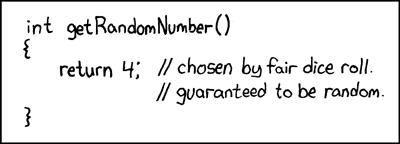# Data loader inconsistent with same path?

I am training a convolutional neural network using fastai. I use a ImageDataLoaders to fetch data from my directory. However, I notice that results of my confusion matrix change if I use another data loader object with exactly the same arguments.
It doesn’t make sense for me since data are already spllitted in train/dev/test sets.

Is that expected behavior ?

My data loader is defined as follow:

``````data = ImageDataLoaders.from_folder(data_directory, ds_tfms=batch_tfms, size=16)
``````

My model is trained as follow:

``````lr = 3e-4
learner = vision_learner(data, arch=resnet34, metrics=accuracy)
learner.freeze()
learner.fit_one_cycle(10,slice(lr),cbs=[ShowGraphCallback()])
``````

The classification result on train set is obtained with the following code snippet:

``````interpret = ClassificationInterpretation.from_learner(learner,dl=data.train)
interpret.plot_confusion_matrix()
``````

The problem happens if I do this again :

``````data2 = ImageDataLoaders.from_folder(data_directory, ds_tfms=batch_tfms, size=16)
interpret = ClassificationInterpretation.from_learner(learner,dl=data2.train)
interpret.plot_confusion_matrix()
``````

What happens if you reset the random seed to the same value prior to both “data =” and “data2 =” ?
Or in other words…1 Like